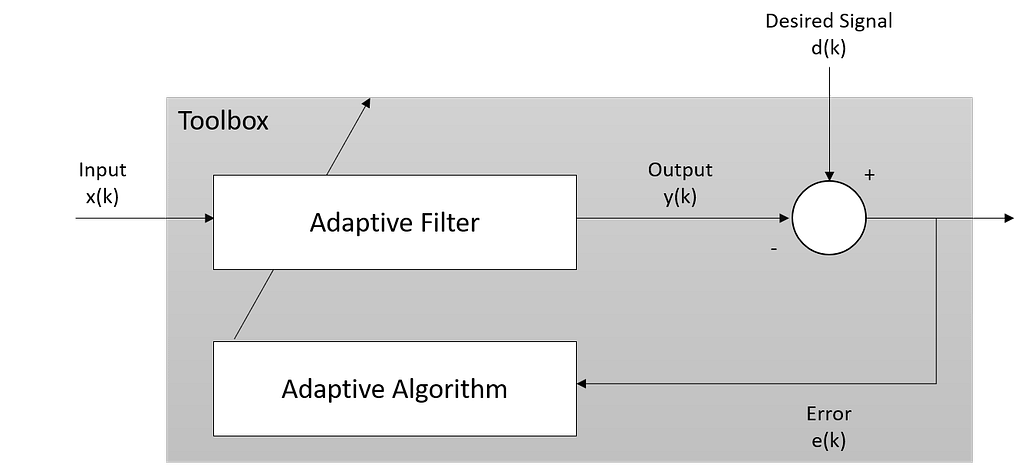Join thousands of AI enthusiasts and experts at the Learn AI Community.

## PublicationMachine Learning

# Time Series prediction using Adaptive filtering

Last Updated on January 6, 2023 by Editorial Team

Last Updated on July 23, 2020 by Editorial Team

### Time Series Prediction using Adaptive Filtering

#### Using adaptive filtering to predict the future time series value in Python

Adaptive filtering is a computational device that attempts to model the relationship between two signals, whose coefficients change with an objective to make the filter converge to an optimal state. The optimization criterion is a cost function, which is most commonly the mean square of the error between the output of the adaptive filter and the desired signal. The mean square error (MSE) will converge to its minimal value, while the filter adapts its coefficients. The figure below demonstrates the simple adaptive filter.

The adaptive filter will try to match the filter output, y(k), with the desired signal, d(k). The adaptive filter will also learn using the error, e(k), and adjust the coefficient. Hence, it is adapted to the new environment, input x(k).

This brings us to the main feature of adaptive filtering, which is it has the real-time capability to adjust the response with the intent to improve its performance (sounds like self-learning, anyone?). The adaptation algorithm is implemented through two methods; gradient method and least square (LMS, RLS algorithm).

### What is it for?

If you have studied any digital signal and processing courses, you will see most of the adaptive filter application on identifying an unknown communications channel, canceling noise or interference, or predicting the future values of a periodic signal.

### So how we can use it in a business setting?

Based on the last example of the digital use case, we can apply the concept to predict future values in real-time settings, for example; stock price prediction. However, predicting the future using this approach requires several key assumptions; the data is either steady or slowly varying over time, and periodic overtime as well.

Accepting these assumptions, the adaptive filter must predict the future values of the desired output based on past input values. Hence, we need to structure the delay in the input signal and feed them to the adaptive filter system.

As stated earlier, the adaptive filter is used to identify and understand the unknown system, we can use this to identify and predict the time series behavior.

### Python implementation

There is a library named padasip in Python, where you can use it to implement adaptive filtering. Check out the library at the following link.

#### Simple implementation example

Let’s take a look at a simple example before using the adaptive filters on time series data. Assume we have the following equation:

We can then prepare the input, target, and run through the filter (using LMS, in this example). For additional sources of filter algorithm, please visit here.

After we run f.run(d, x) , we will get the prediction (y), error (e), and the weight of each iteration (w). We can see that the first several iterations, the filter cannot accurately predict the target but as the higher iterations, the filter starts to adapt and predict closely to target values.The comparison between actual and predicted value (upper figure), the error for each iteration (lower figure)

#### Implementation using NLMS on stock price data

Now let’s try to predict the Uniqlo’s closing stock price (from 2012–2016).

We will start by building the filter using the first 1000 data points with 5 lagged data points for each prediction iteration. Three values of mu have been tested, sample code with best mu (minimum error) has been shown below.

We can see how the filter required several (around 60) iterations to adjust the weights to fit the data. During this phase, we are also getting the filter for further usage. Snippet code below shows how we can use them in a production environment.

From the code, we get the best filter from the previous step and use it to predict the new data points (line 18). After the prediction is made, we then use the adapt the method of the filter to adjust the weights (line 20). The output and error have been visualized below.

#### Endnote

In this example, I demonstrate how we can use the adaptive filter on time series data to predict the future value. There is a lot of filter and algorithm to try for the adaptive filter. Proper filter type may need to view and select for the given problem you have. Hopefully, this introduces you to the adaptive filter and its basic implementation onto the real-world scenario.

You can view the full notebook and the sample dataset on my Github (or click here to view the notebook directly) and feel free to connect with me on LinkedIn.Time Series prediction using Adaptive filtering was originally published in Towards AI — Multidisciplinary Science Journal on Medium, where people are continuing the conversation by highlighting and responding to this story.

Published via Towards AI